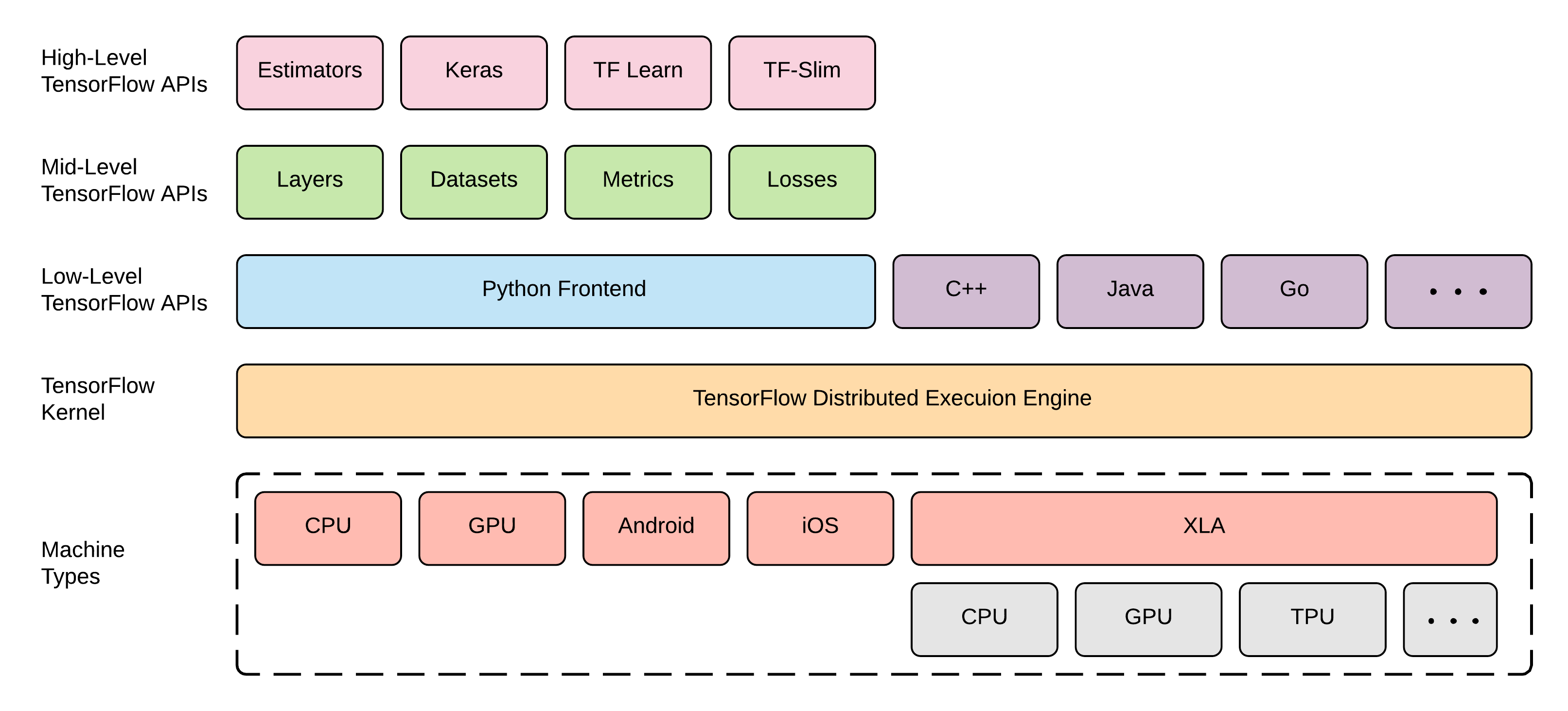# 給 TensorFlow 初學者或害怕複雜 API 的人的學習建議

## 前言## 入門建議

# 定義 model
model = tf.keras.models.Sequential([
tf.keras.layers.Flatten(input_shape=(28, 28, 1)),
tf.keras.layers.Dense(128,activation='relu'),
tf.keras.layers.Dense(10, activation='softmax')
])

# 指定 loss、optimizer
model.compile(
loss='sparse_categorical_crossentropy',
optimizer=tf.keras.optimizers.Adam(0.001),
metrics=['accuracy'],
)

# 開始 train model：使用 ds_train dataset，跑 6 個 epoch
#                  使用 ds_test 做 validation
model.fit(
ds_train,
epochs=6,
validation_data=ds_test,
)


# 定義 model
def neural_net(x):
layer_1 = tf.add(tf.matmul(x, weights['h1']), biases['b1'])
layer_2 = tf.add(tf.matmul(layer_1, weights['h2']), biases['b2'])
out_layer = tf.matmul(layer_2, weights['out']) + biases['out']
return out_layer

# 建立 model 的輸出
logits = neural_net(X)
prediction = tf.nn.softmax(logits)

# 指定 loss、optimizer
loss_op = tf.reduce_mean(tf.nn.softmax_cross_entropy_with_logits(
logits=logits, labels=Y))
optimizer = tf.train.AdamOptimizer(learning_rate=learning_rate)

# 把 optimizer 跟 loss_op 接起來（嗯，已經開始有點不直覺了，有 predictions、又有 loss_op、現在還要多一個 train_op，怎麼那麼多層）
train_op = optimizer.minimize(loss_op)

# 指定初始化 model 參數的負責人
init = tf.global_variables_initializer()

# 準備開始 training（hmmm....session 是啥？）
with tf.Session() as sess:

# 初始化 model 參數
sess.run(init)

for step in range(1, num_steps+1):
batch_x, batch_y = mnist.train.next_batch(batch_size)
# 實際 training
sess.run(train_op, feed_dict={X: batch_x, Y: batch_y})


## 理解 computational graph## 了解其他 API 的定位

TF 的其中一個強大之處，在於他有很多 API，但這也大大增加了這個工具的複雜度，讓學習曲線大幅變陡。而為了在學習新的 API、或是上網找各種解答時不混亂，心中有個大地圖是非常重要的：## 總結